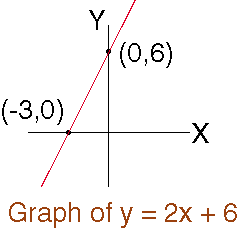Name: Cassandra Who is asking: Student Level: Secondary Question: My book says to find where the X and Y intercept, i dont understand who to do this problem. Can you please help here is the problem. it didnt quite explain the instructions. 3x=y Thank You Hi Cassandra, "Find the X and Y intercepts" means find the points where the graph crosses the X-axis and the Y-axis. I want to first consider a different equation, y = 2x + 6, with the same instructions, find the X and Y intercepts.    If you substitute x = 0 in the equation y = 2x + 6 you find y = 6. Thus the point (0,6) is on the graph. This is the point where the graph crosses the Y-axis and hence the Y-intercept is 6.    If you substitute y = 0 in the equation y = 2x + 6 you find x = -3. Thus the point (-3,0) is on the graph. Therefore the X-intercept is -3.If we now consider your original equation, y = 3x then substituting x = 0 gives y = 0 and thus the Y-intercept is 0. Likewise substituting y = 0 gives x = 0 and thus the X-intercept is also 0. To see why this is true plot the graph of y = 3x. Cheers, Penny Go to Math Central